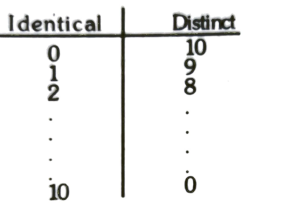Combinations
Question

# The number of ways of selecting 10 objects out 10 identical and 20 distinct objects is

Moderate
Solution

##No. of ways  ${=}^{20}{\mathrm{C}}_{10}{+}^{20}{\mathrm{C}}_{9}{+}^{20}{\mathrm{C}}_{8}+\dots {+}^{20}{\mathrm{C}}_{0}=\frac{{2}^{20}{+}^{20}{\mathrm{C}}_{10}}{2}$

Get Instant Solutions